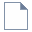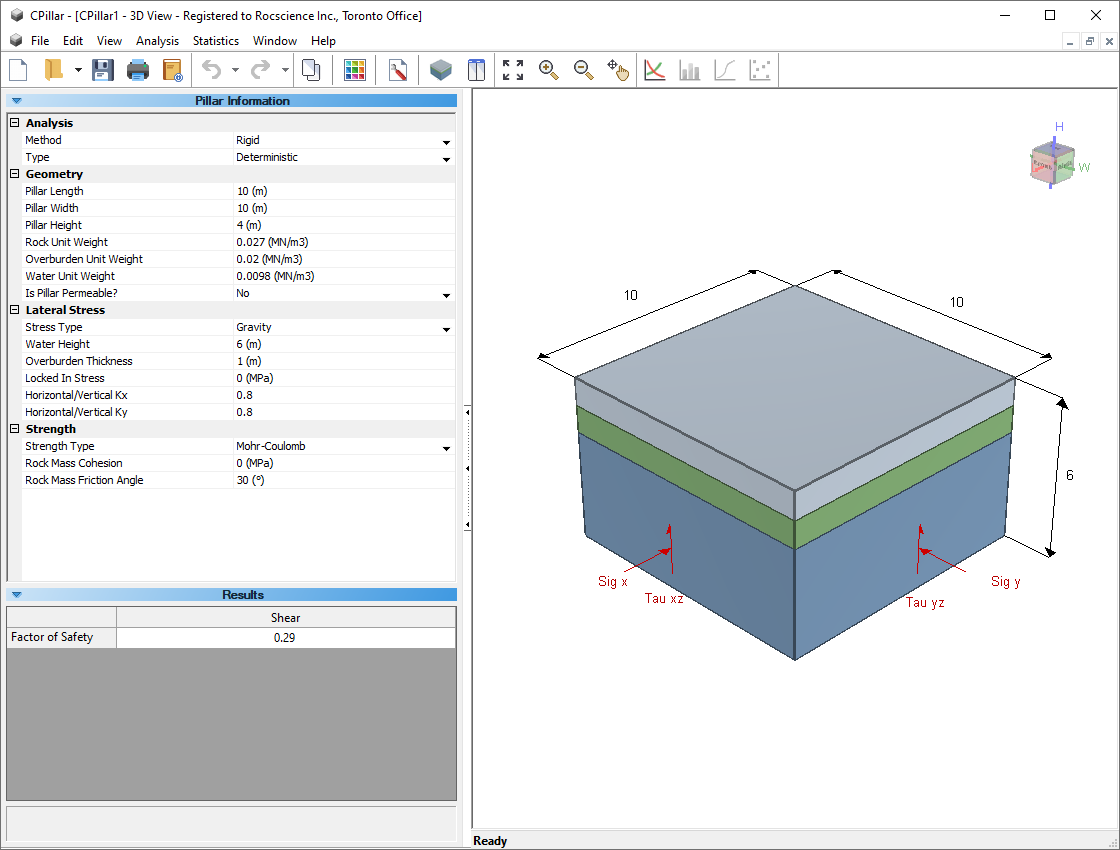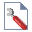The Rocscience International Conference 2021 Proceedings are now available. Read Now

# 2 - Elastic Analysis of Rectangular Roof Beam

In Tutorial 01 - CPillar Quick Start (Rigid Analysis of Square Pillar), you learned about the two main analysis types in CPillar: Deterministic, and Probabilistic, and how to run a Deterministic analysis (the default). In this tutorial, you will learn how to run a Probabilistic analysis.

In a Probabilistic analysis, statistical input data can be entered to account for uncertainty in geometry, lateral stress, and strength values. The result is a distribution of Factors of Safety, from which a Probability of Failure is calculated. To learn more about Probabilistic Analysis, see Overview of Probabilistic Analysis in CPillar.

Topics Covered in this Tutorial:

• Project Settings
• Random Variables
• Roof Beam Model
• Probabilistic Analysis
• Elastic Analysis for Rectangular Pillar
• Hoek-Brown Strength Criterion
• Constant Lateral Stress
• Failure Modes
• Histogram Plot

Finished Product:

The finished product of this tutorial can be found in the Tutorial 2 Elastic Analysis of Rectangular Roof Beam (Long Excavation).cpil5 file, located in the Examples > Tutorials folder in your CPillar installation folder.

## 1.0 Introduction

This model represents a roof beam (10 x 100 m) with a thickness of 1 m. There is 1 m of overburden having the same density as the beam.

The lateral stress is defined as a constant. Lateral effective stresses of 2 ± 0.5 MPa in both the x and y directions will be used.

## 2.0 Creating a New File

If you have not already done so, run the CPillar program by double-clicking the CPillar icon in your installation folder or by selecting Programs > Rocscience > CPillar > CPillar in the Windows Start menu.

When the program starts, a default model is automatically created. If you do NOT see a model on your screen:Select: File > New

Whenever a new file is created, the default input data forms valid pillar geometry, as shown in the image below.If the CPillar application window is not already maximized, maximize it now so that the full screen is available for viewing the model. You will have a 3D pillar displayed on the screen in isometric orientation.

## 3.0 Project Settings

The Project Settings dialog allows you to configure the main analysis parameters for your model, such as Units, Analysis Type, and Sampling Method. Select Project Settings on the toolbar or on the Analysis menu.Select: Analysis > Project Settings### 3.1 ANALYSIS TYPE

BY DEFAULT A DETERMINISTIC ANALYSIS IS SELECTED FOR A NEW FILE.

1. Select the General tab.
2. Change the Analysis Type to Probabilistic.

### 3.2 UNITS

IN THIS TUTORIAL WE ARE USING METRIC (MPA) UNITS, SO MAKE SURE THE METRIC, STRESS AS MPA OPTION IS SELECTED FOR UNITS IN THE PROJECT SETTINGS GENERAL TAB (DEFAULT SETTING).

### 3.3 SAMPLING AND RANDOM NUMBERS

THE SAMPLING METHOD DETERMINES HOW THE STATISTICAL DISTRIBUTION FOR THE RANDOM INPUT VARIABLES WILL BE SAMPLED. THE DEFAULT SETTINGS ARE SAMPLING METHOD = LATIN HYPERCUBE AND NUMBER OF SAMPLES = 5,000. FOR MORE HELP, SEE Sampling in CPillar Project Settings.

### 3.4 PROJECT SETTINGS

Select the Project Summary tab and enter CPillar Elastic Analysis of Rectangular Roof Beam Tutorial as the Project Title.NOTE: You can have Project Summary information appear on analysis results printouts by setting up a header or footer through Page Setup on the File menu.

• Click OK to close the Project Settings dialog.

## 4.0 Probabilistic Input Data

Autocompute is turned ON by default when performing a Probabilistic Analysis. Autocompute will automatically compute the model every time a change is made in Pillar Information. Let's disable it for now to save on computing time when entering input data.

1. Select: Analysis > Autocompute
2. Uncheck Autocompute for probability analysis3. Click OK.

In CPillar, the entirety of the input parameters are entered in the Pillar Information section of the Sidebar. The Pillar Information section is organized under four headings: Analysis, Geometry, Lateral Stress, and Strength. To change a parameter, click on the value and enter the new value or select from the dropdown as necessary. The model will reflect any changes, immediately.

### 4.1 ANALYSIS

Set up the Analysis:

1. Analysis Method = Elastic
2. Analysis Type = Probabilistic### 4.2 GEOMETRY

Enter the following mean data for the pillar geometry parameters:

1. Mean Pillar Length = 10 m
2. Mean Pillar Width = 100 m
3. Mean Pillar Height = 1 m
4. Mean Rock Unit Weight = 0.027 MN/m3
5. Mean Overburden Unit Weight = 0.027 MN/m3
6. Water Unit Weight = 0.0098 MN/m3
7. Is Pillar Permeable? = No

### 4.3 LATERAL STRESS

Enter the following mean data for the stress parameters:

1. Stress Type = Constant
2. Mean Water Height = 0 m
3. Mean Overburden Thickness = 1 m
4. Mean Horizontal Sigma x = 2 MPa
5. Mean Horizontal Sigma y = 2 MPa

### 4.4 STRENGTH

Enter the following mean data for the strength parameters:

1. Strength Type = Hoek-Brown
2. Mean Intact UCS = 50 MPa
3. Mean Rock Mass m Value = 0.3
4. Mean Rock Mass s Value = 0.0001
5. Mean Rock Mass Modulus = 5000 MPa

### 4.5 RANDOM VARIABLES

In order to perform a Probabilistic analysis, at least one random variable must be assigned a statistical distribution. The Statistical Distribution is changed by clicking on the distribution iconto the left of the parameter value.

Let's model the following parameters as random variables:

1. Click the distribution iconto the left of Mean Horizontal Sigma x, and set:
2. Distribution = Normal

Std. Dev. = 0.5 MPa

Rel. Min and Rel Max = 3x Std. Dev. (Check 3x std. dev.)3. Click the distribution iconto the left of Mean Horizontal Sigma y, and set:
4. Distribution = Normal

Std. Dev. = 0.5 MPa

Rel. Min and Rel Max = 3x Std. Dev. (Check 3x std. dev.)

5. Click the distribution iconto the left of Intact UCS, and set:
6. Distribution = Normal

Std. Dev. = 5

Rel. Min and Rel Max = 3x Std. Dev. (Check 3x std. dev.)